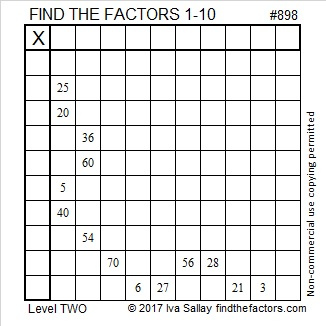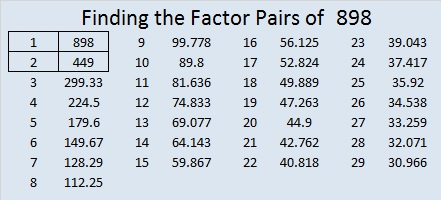# 898 and Level 2

27² + 13² = 898. That means that 898 is the hypotenuse of a Pythagorean triple:

• 560-702-898, which is 2 times (280-351-449).

898 reads the same way frontwards and backwards so it is a palindrome in base 10.

It is also palindrome 747 in BASE 11 because 7(11²) + 4(11) + 7(1) = 898

AND it is palindrome 1G1 in BASE 23 (G is 16 in base 10) because 1(23²) + 16(23) +1(1) =898.Print the puzzles or type the solution on this excel file: 10-factors-897-904

• 898 is a composite number.
• Prime factorization: 898 = 2 × 449
• The exponents in the prime factorization are 1 and 1. Adding one to each and multiplying we get (1 + 1)(1 + 1) = 2 × 2 = 4. Therefore 898 has exactly 4 factors.
• Factors of 898: 1, 2, 449, 898
• Factor pairs: 898 = 1 × 898 or 2 × 449
• 898 has no square factors that allow its square root to be simplified. √898 ≈ 29.9666